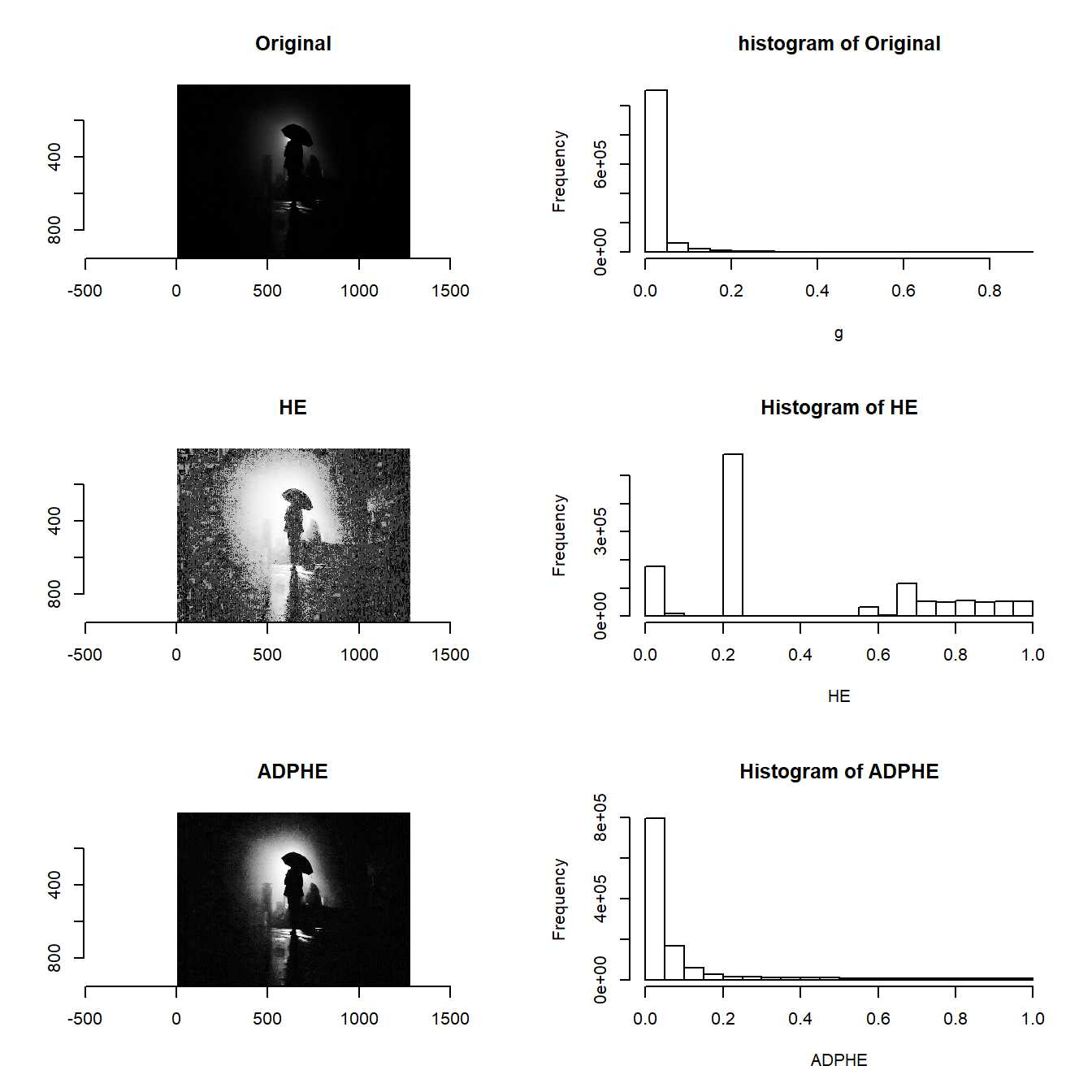Histogram equalization (HE) is a widely used technique for contrast enhancement of images.

However, HE sometimes causes over-enhancement of background noise, whereas the contrast of objects is not enhanced well.

Double plateaus histogram equalization (DPHE) was proposed for overcoming this problem.

DPHE modifies original histogram as shown below, then equalizes the modified histogram.

$H_{m}(g) = \begin{cases} T_{UP} & h(g) >= T_{UP} \\ h(g) & T_{DOWN} < h(g) < T_{UP} \\ T_{DOWN} & h(g) <= T_{DOWN} \end{cases}$ where $$g$$ is the gray level, $$h(g)$$ is the original histogram, and $$H_{m}(g)$$ is the modified histogram.

The paramters, $$T_{DOWN}$$ and $$T_{UP}$$, are key parameters and its optimal values are scene specific.

Tuning the parameters for each scene are annoying.

That’s why adaptive double plateaus histogram equalization (ADPHE), which computes the parameters automatically, was proposed.

We can use the functions as shown below.

devtools::install_github("ShotaOchi/imagerExtra")
library(imagerExtra)
hist(ADPHE, main = "Histogram of ADPHE")